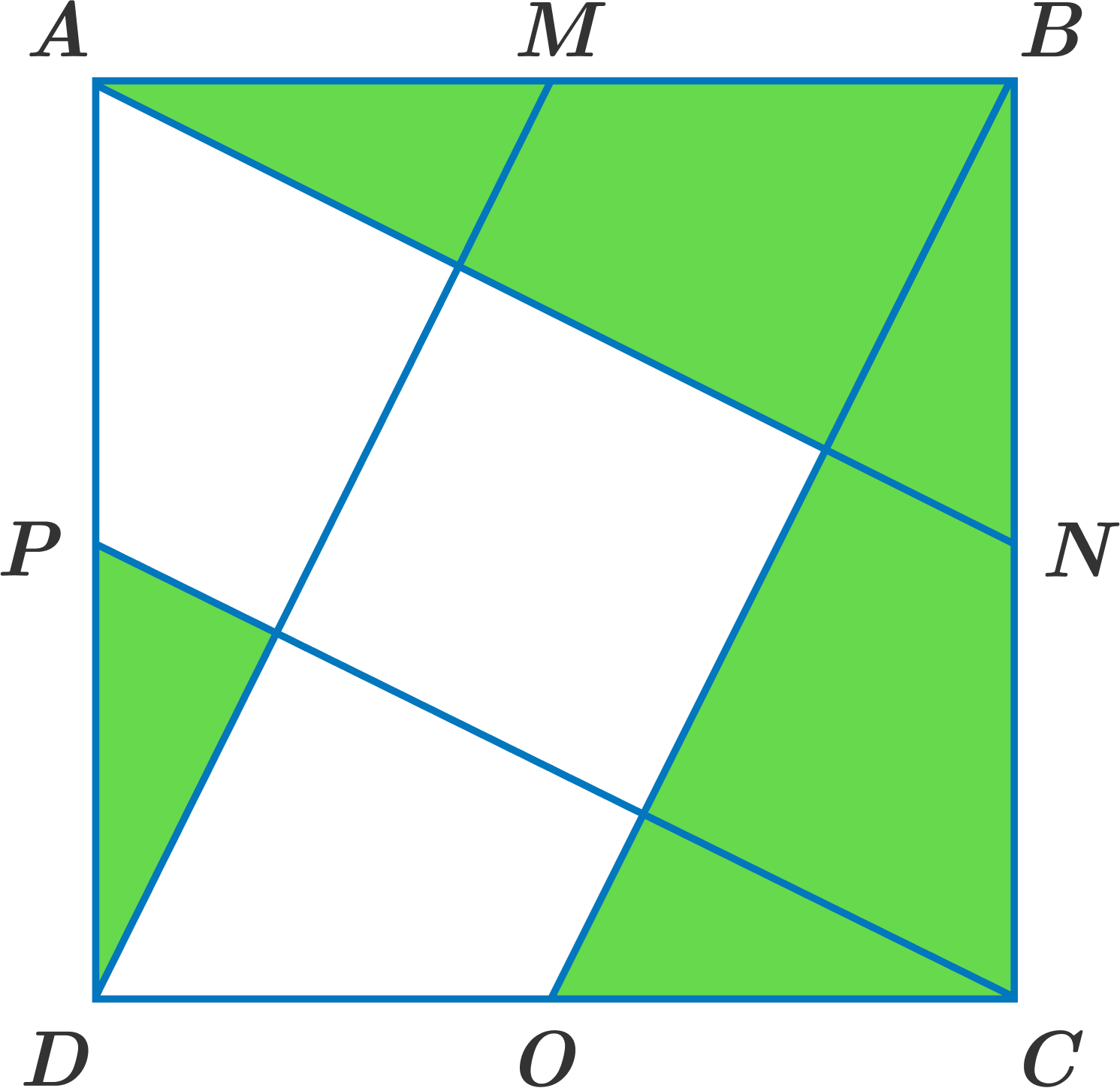# Tricky Colors

Geometry Level 1$ABCD$ is a square with $M$, $N$, $O$, and $P$ being the midpoints of their respective sides.

Which of the following has a larger area inside the square: the shaded (green) region or the non-shaded (white) region?

×